# Smart Refrigrator

Sends an Email using Mailgun services when temperature is not within the prescribed range. Sends an SMS when fridge is opened using TwiIio

IntermediateProtip8 hours79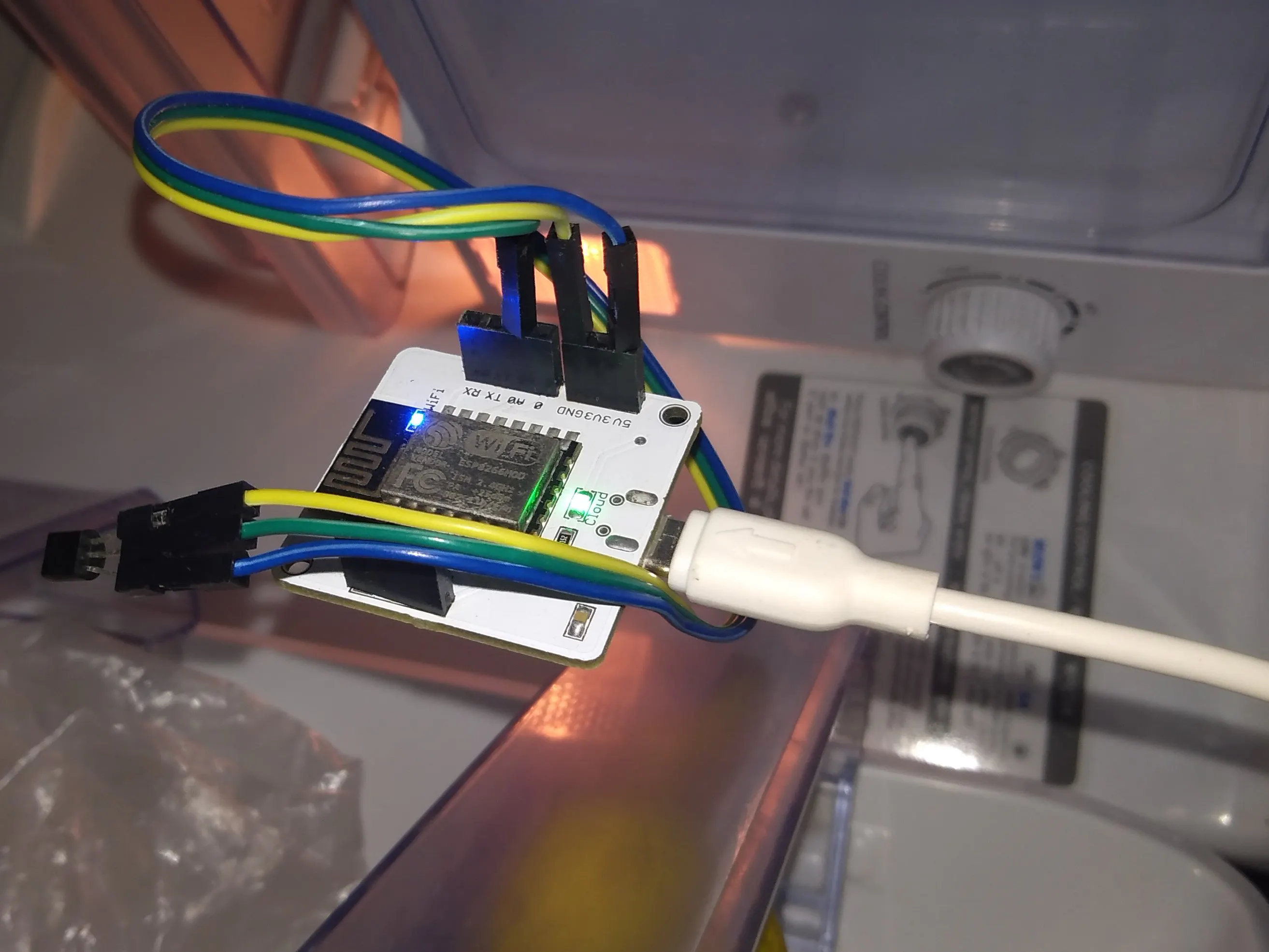## Things used in this project

### Hardware components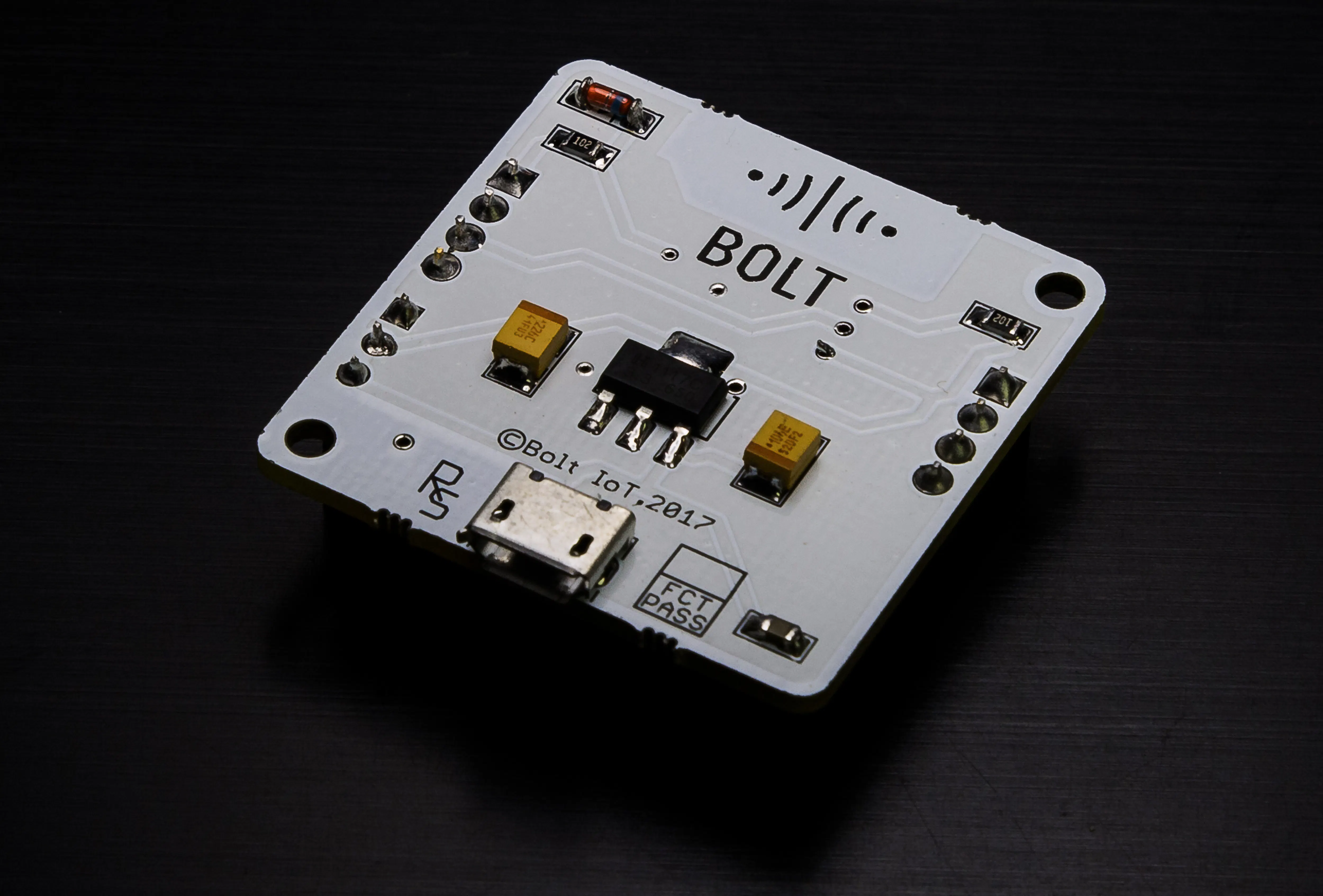Bolt IoT Bolt WiFi Module
×1Jumper wires (generic)
×3Temperature Sensor
×1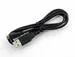USB-A to Micro-USB Cable
×1

### Software apps and online services

 Mailgun To receive email when temperature is not in desired range.Twilio SMS Messaging API To receive SMS when someone opens the fridge.Bolt IoT Bolt Cloud To collect the data from wifi module and for prediction.

## Custom parts and enclosures

### Running the python code

by using
python3 anomaly_detection.py

Below are the screenshots of programme code, output,message,amd email which i got.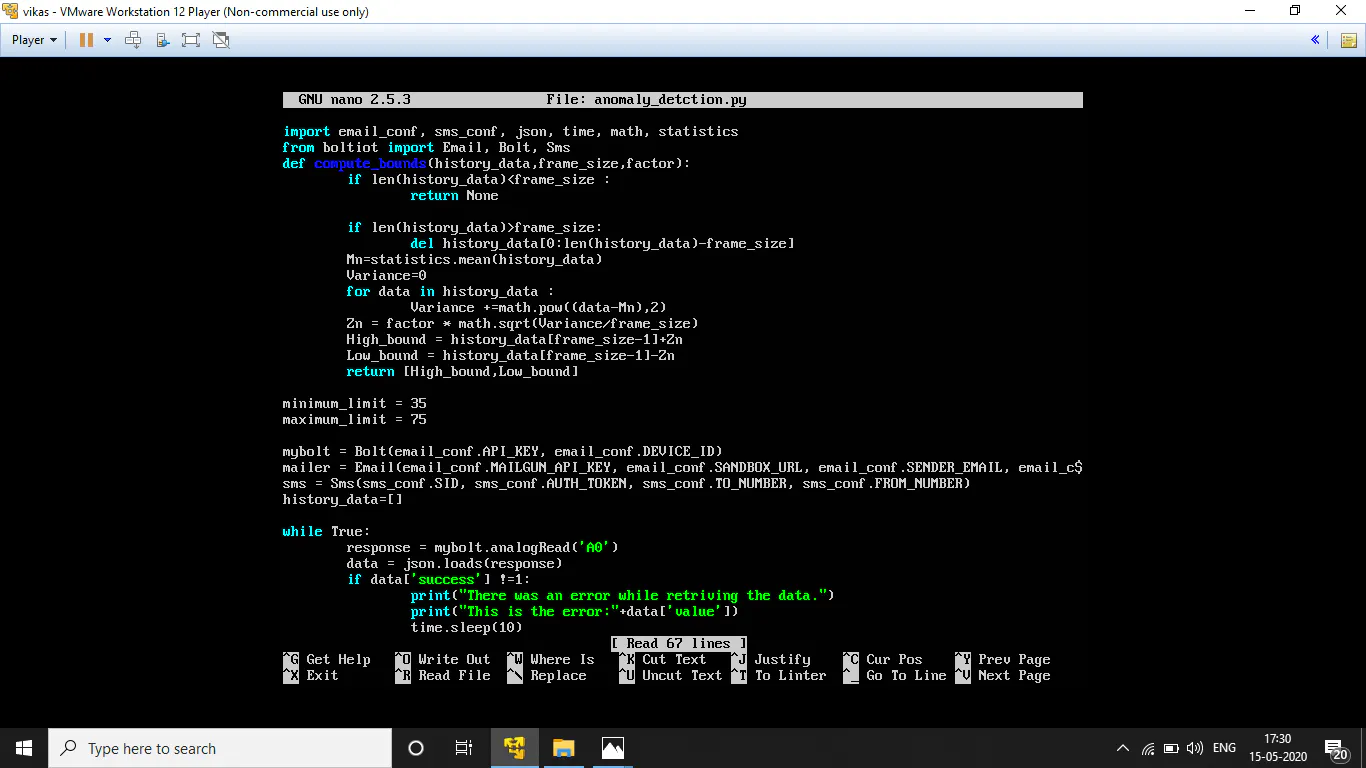### 2_9EmtINePpX.png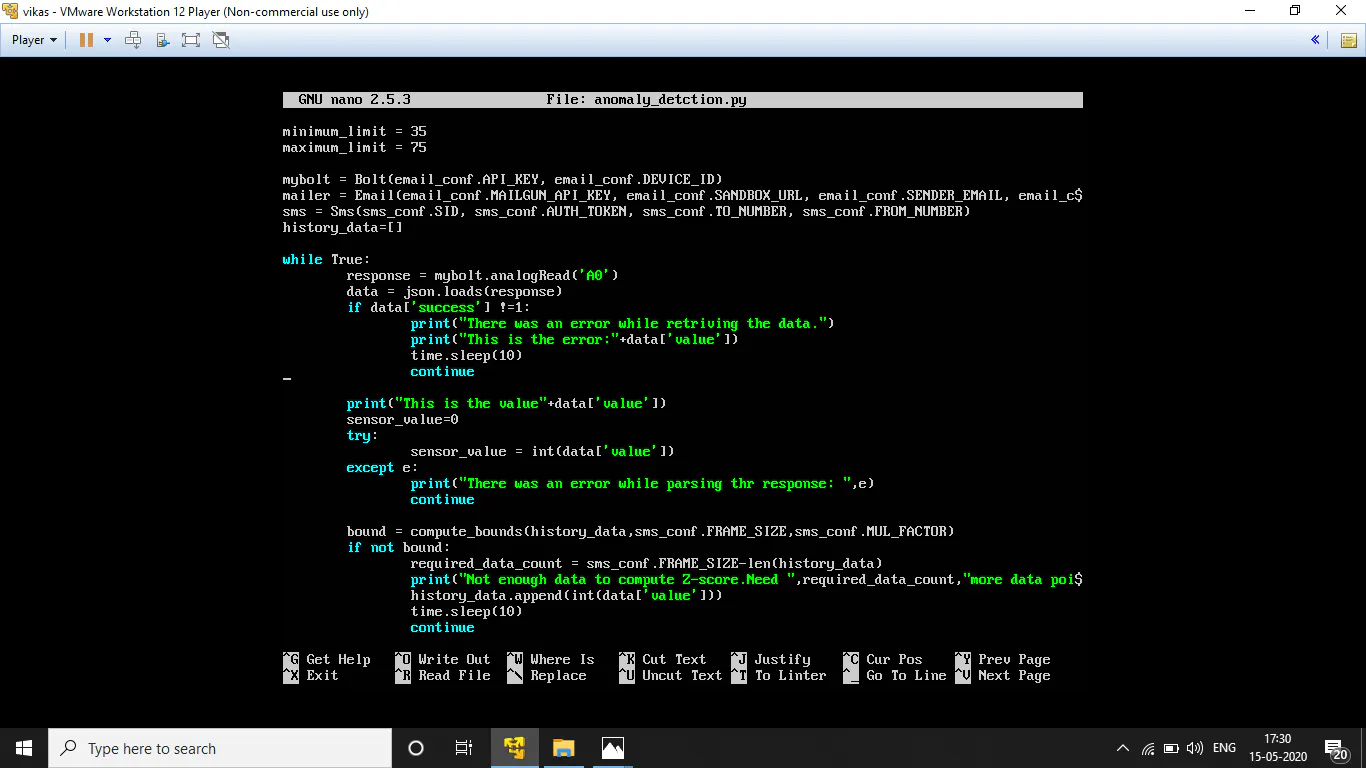### 3_YLVuKPRt6V.png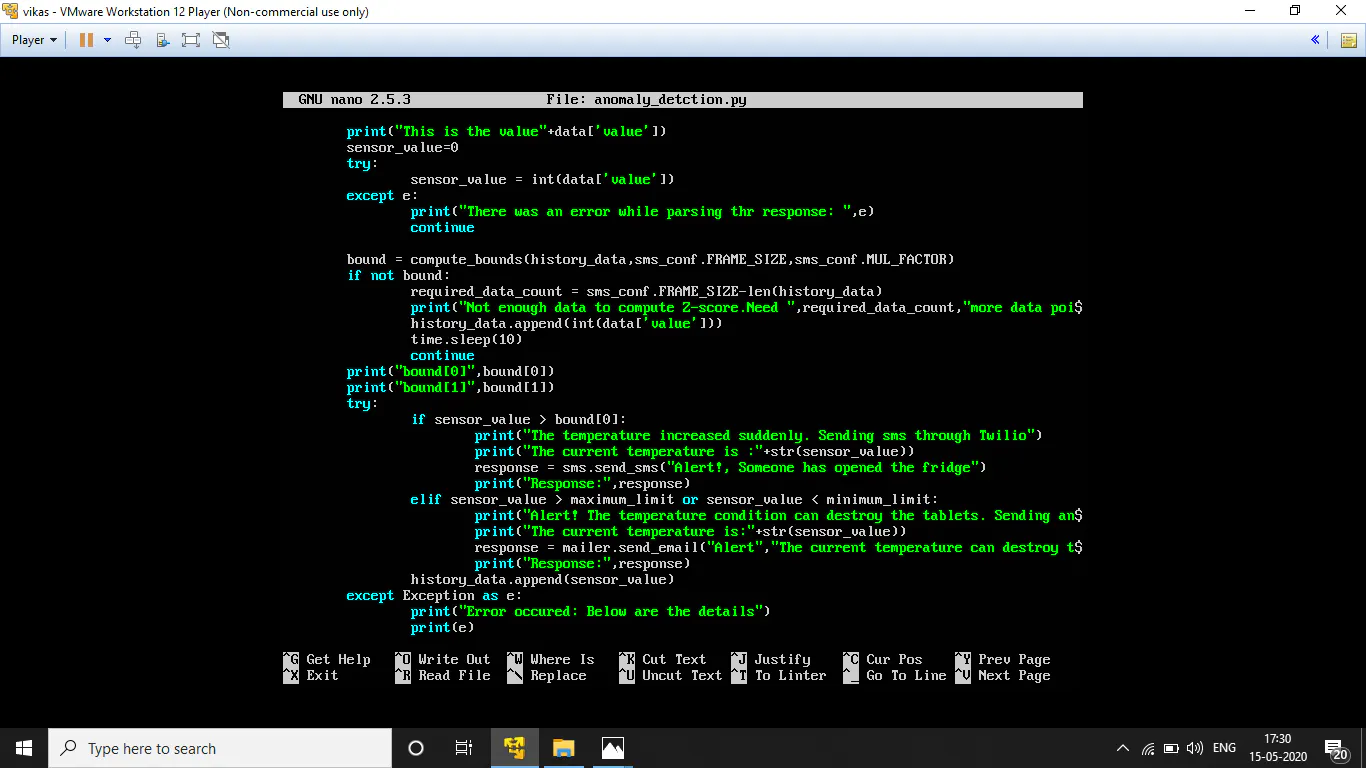### 4_NYzV5n6ZDz.png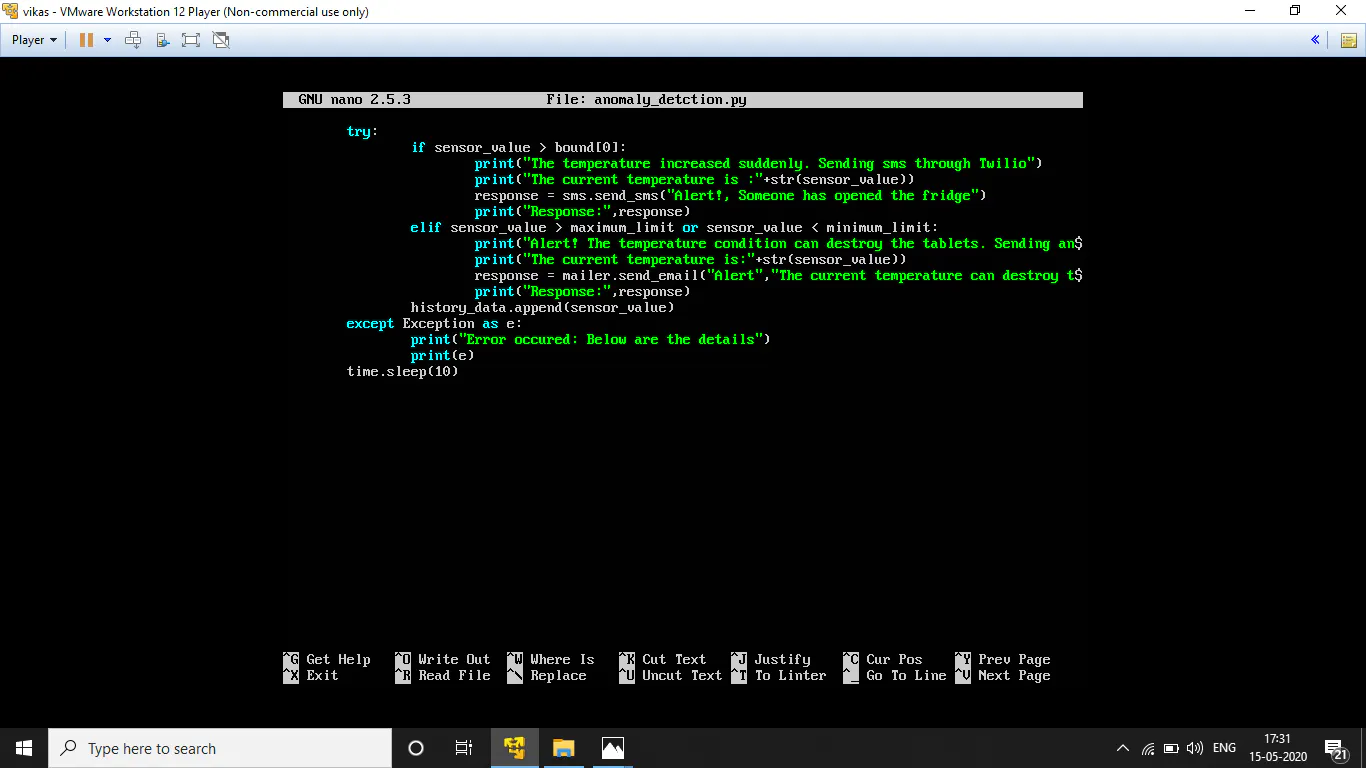### 5_kvRnWRHVok.png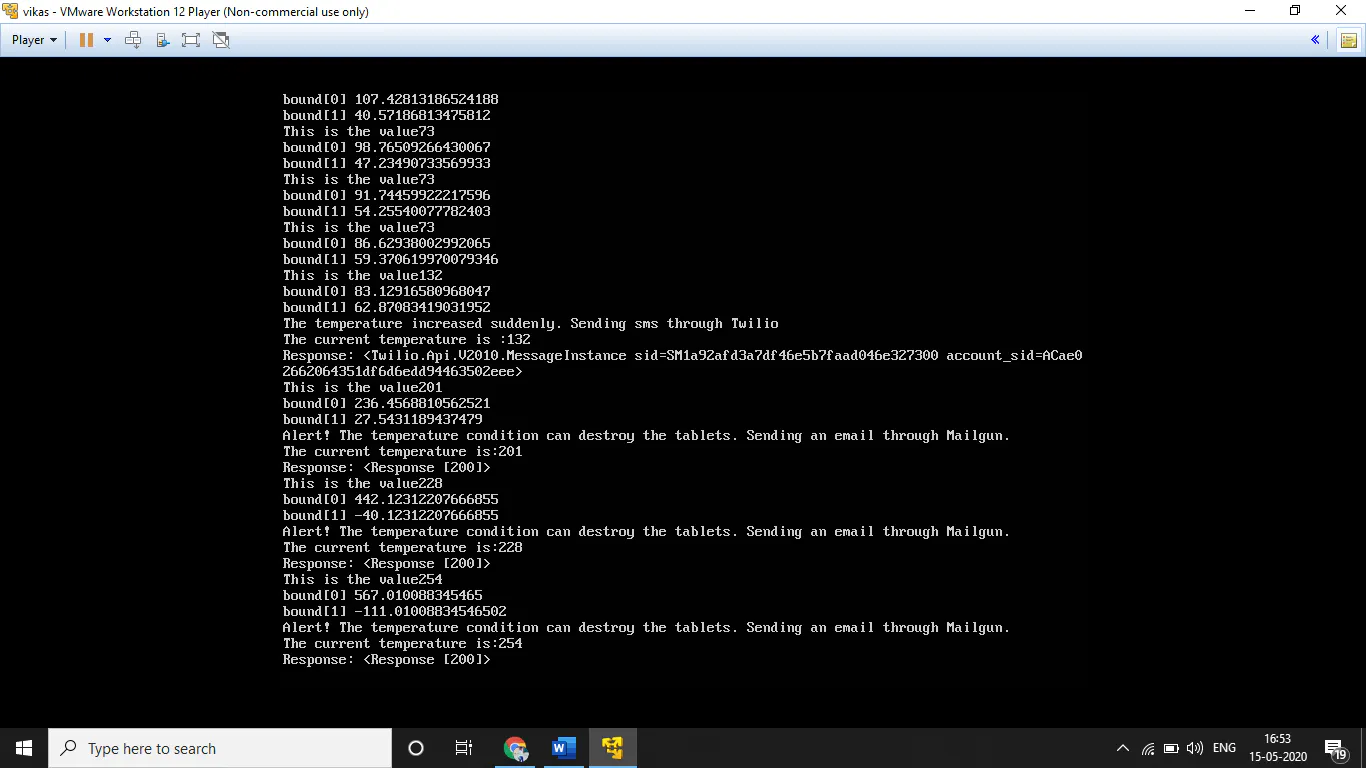### 7_(1)_q6ZSCR9bH7.png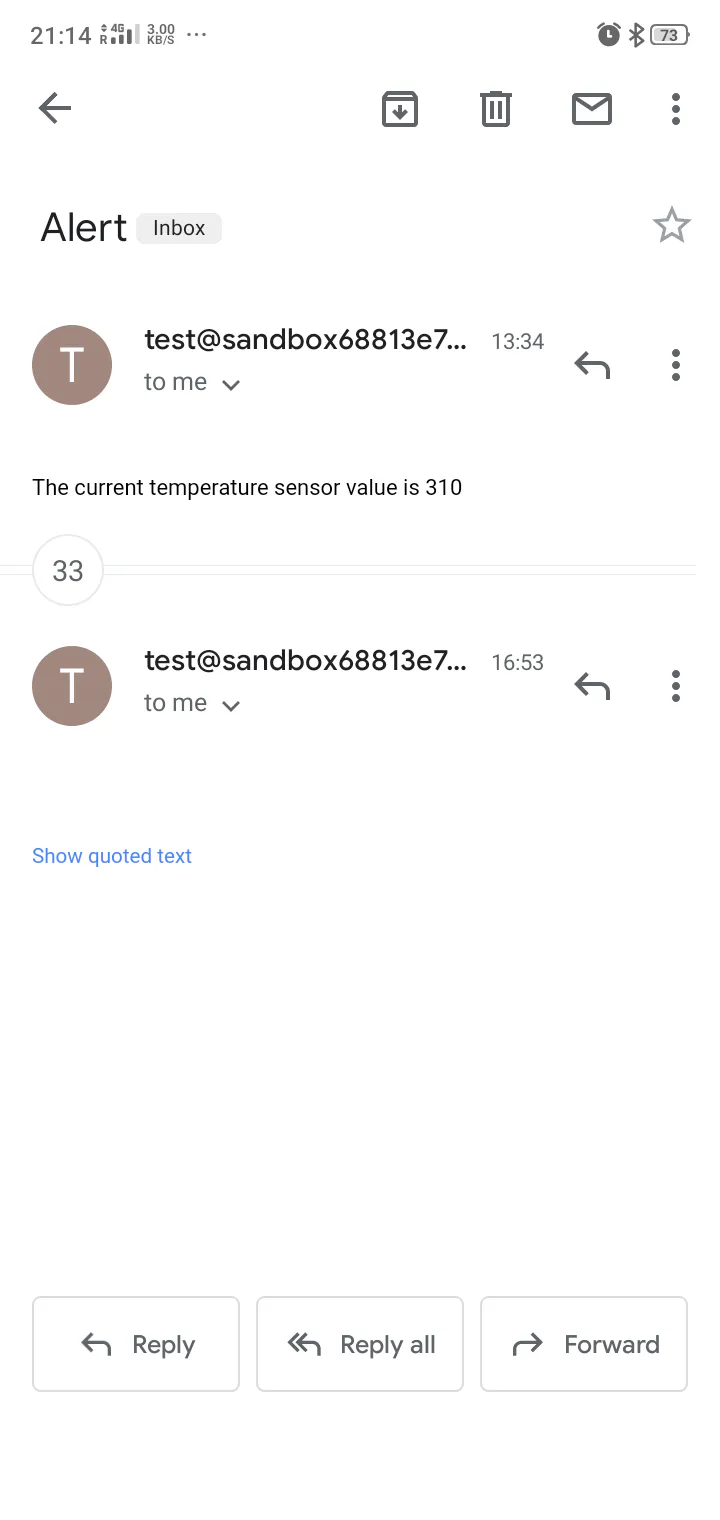### 7_(2)_Aq9x4dDywO.png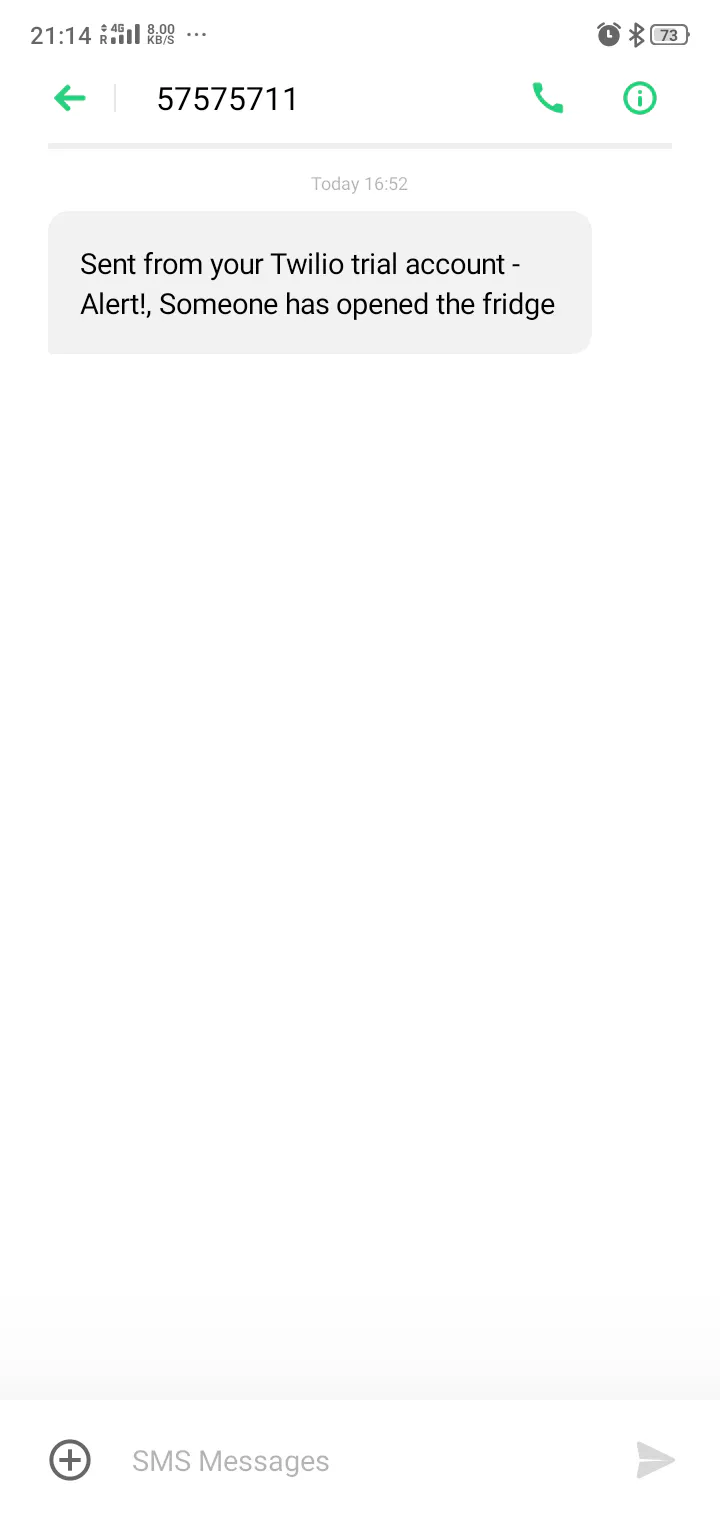## Schematics

### Circuit

Image of circuit which i have connected.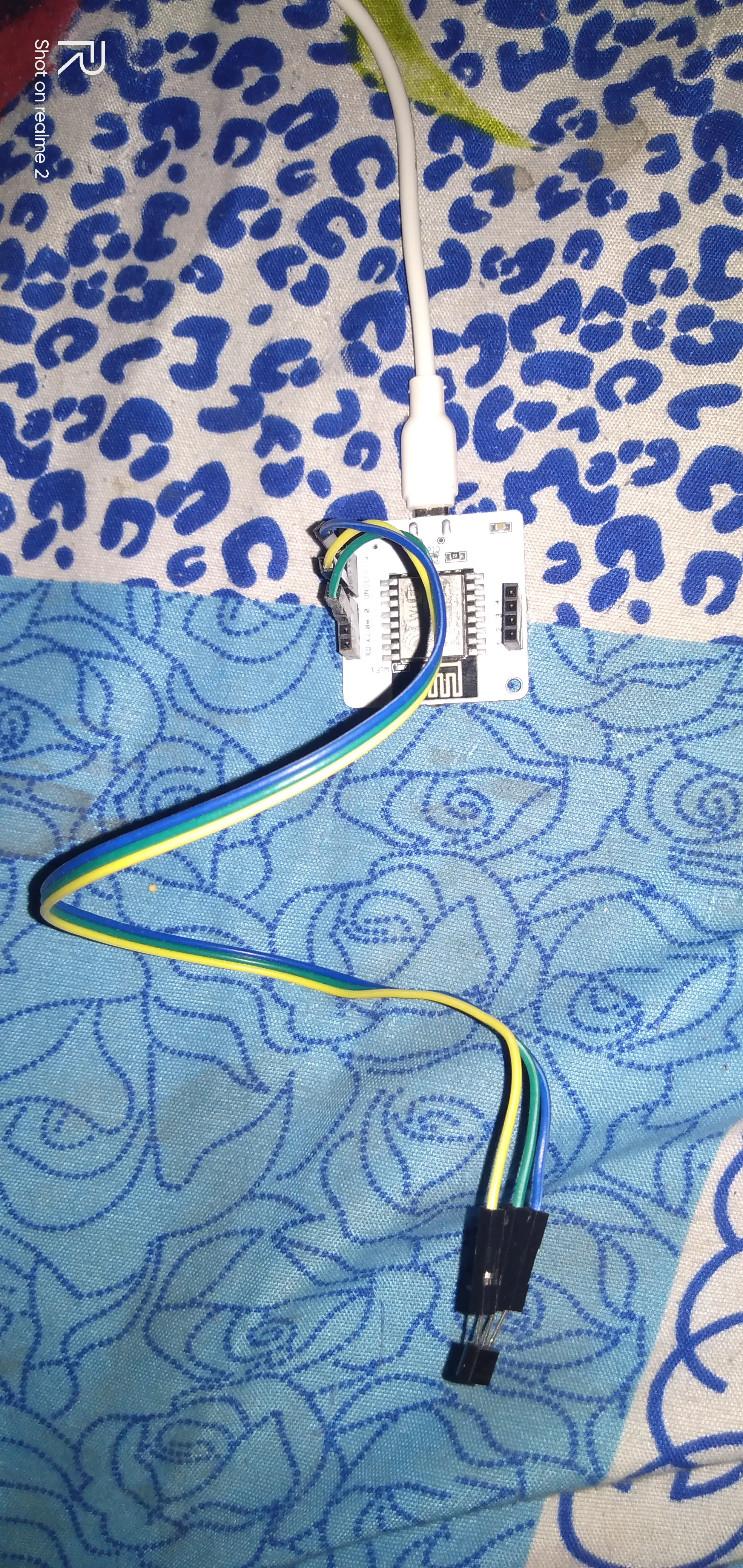## Code

### Code

Python
We have to import our ( sms_conf & email_conf ) files which have all the credentials, json and time.
Also we import our Bolt python library which will let us fetch the data stored in Bolt Cloud and then based on value send Email and SMS.
The math and statistics libraries will be required for calculating the Z-score and the threshold boundaries.
The following lies code helps define a function which calculates the Z-score and the using the Z-score calculates the boundaries required for anomaly detection.
The above line helps define a function, which takes 3 input variables: hisotry_data, frame_size and factor.
The above code checks whether enough data has been accumulated to calculate the Z-score, and if there is too much data, then the code deletes the older data.
The above code calculates the mean (Mn) value of the collected data points.
This code helps to calculate the Variance of the data points
Here we calculate the Z score (Zn) for the data and use it to calculate the upper and lower threshold bounds required to check if a new data point is normal or anomalous.
Now we will initialize two variables which will store minimum and maximum threshold value. You can initialize any minimum and maximum integer limits to them.
This would send an alert if the temperature reading goes below the minimum limit or goes above the maximum limit.
The above code will automatically fetch your API key and Device ID that you have initialized in email_conf.py file.
The above code will automatically fetch your MAILGUN_API_KEY, SANDBOX_URL, SENDER_EMAIL and RECIPIENT_EMAIL that you have initialized in email_conf.py file. Make sure you have entered the correct values in email_conf.py file.
To collect data and send SMS alerts. Here we also initialize an empty list with the name 'history_data' which we will use to store older data, so that we can calculate the Z-score.
The following while loop contains the code required to run the algorithm of anomaly detection.
```import email_conf, sms_conf, json, time, math, statistic
from boltiot import Email, Bolt, Sms
def compute_bounds(history_data,frame_size,factor):
if len(history_data)<frame_size :
return None
if len(history_data)>frame_size :
del history_data[0:len(history_data)-frame_size]
Mn=statistics.mean(history_data)
Variance=0
for data in history_data :
Variance += math.pow((data-Mn),2)
Zn = factor * math.sqrt(Variance / frame_size)
High_bound = history_data[frame_size-1]+Zn
Low_bound = history_data[frame_size-1]-Zn
return [High_bound,Low_bound]
minimum_limit = 35
maximum_limit = 75
mybolt = Bolt(email_conf.API_KEY, email_conf.DEVICE_ID)
mailer = Email(email_conf.MAILGUN_API_KEY, email_conf.SANDBOX_URL, email_conf.SENDER_EMAIL, email_conf.RECIPIENT_EMAIL)
sms = Sms(conf.SID, conf.AUTH_TOKEN, conf.TO_NUMBER, conf.FROM_NUMBER)
history_data=[]
while True:
if data['success'] != 1:
print("There was an error while retriving the data.")
print("This is the error:"+data['value'])
time.sleep(10)
continue
print ("This is the value "+data['value'])
sensor_value=0
try:
sensor_value = int(data['value'])
except e:
print("There was an error while parsing the response: ",e)
continue
bound = compute_bounds(history_data,conf.FRAME_SIZE,conf.MUL_FACTOR)
if not bound:
required_data_count=conf.FRAME_SIZE-len(history_data)
print("Not enough data to compute Z-score. Need ",required_data_count," more data points")
history_data.append(int(data['value']))
time.sleep(10)
continue

print("bound",bound)
print("bound",bound)
try:
if sensor_value > bound :
print ("The Temperature increased suddenly. Sending a sms through Twilio.")
print ("The Current temperature is: "+str(sensor_value))
response = sms.send_sms("Alert! Someone has opened the fridge door")
print("Response :",response)
elif sensor_value > maximum_limit or sensor_value < minimum_limit:
print("Alert! The temperature condition can destroy the tablets. Sending an email through Mailgun.")
print ("The Current temperature is:" +str(sensor_value))
response = mailer.send_email("Alert!","The current temperature can destroy the tablets.")
print("Response:",response)
history_data.append(sensor_value);
except Exception as e:
print ("Error",e)
time.sleep(10)
```

## Credits

### VIKAS KUMAR

1 project • 0 followers